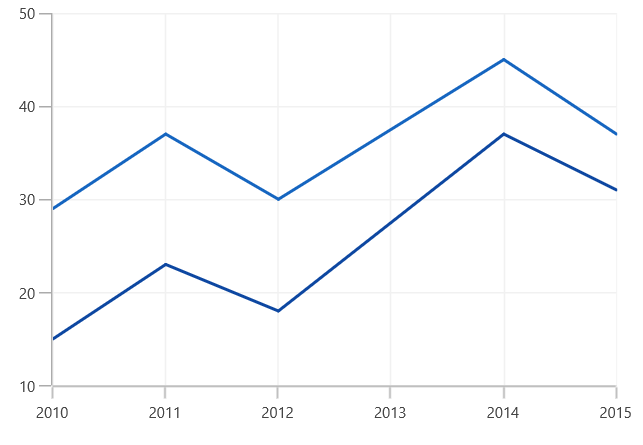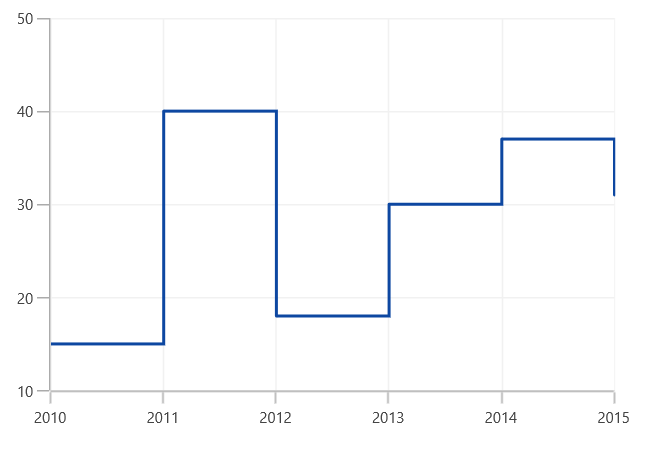# Line and StepLine in WinUI Chart

14 Apr 20211 minute to read

## Line

Line series join points on a plot by straight lines, showing data trends at equal intervals. The following code example explains how to create a simple `LineSeries` using given data.

``````<chart:LineSeries XBindingPath="Year" ItemsSource="{Binding Data}" YBindingPath="Value"/>

<chart:LineSeries XBindingPath="Year" ItemsSource="{Binding Data}" YBindingPath="Value1"/>``````
``````LineSeries series1 = new LineSeries()
{

ItemsSource = new ViewModel().Data,

XBindingPath = "Year",

YBindingPath = "Value",

};

LineSeries series2 = new LineSeries()
{

ItemsSource = new ViewModel().Data,

XBindingPath = "Year",

YBindingPath = "Value1",

};

chart.Series.Add(series1);

chart.Series.Add(series2);``````## Step Line

`StepLineSeries` plots horizontal and vertical lines to connect data points resulting in a step line progression. The following code illustrates how to initialize the `StepLineSeries` in chart.

``````<chart:StepLineSeries ItemsSource="{Binding Data}" XBindingPath="XValue" YBindingPath="YValue">
</chart:StepLineSeries>``````
``````StepLineSeries stepLine = new StepLineSeries();

stepLine.ItemsSource = new ViewModel().Data;

stepLine.XBindingPath = "XValue";

stepLine.YBindingPath = "YValue";

SteplineChart.Series.Add(stepLine);``````